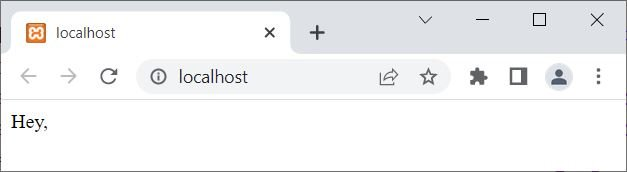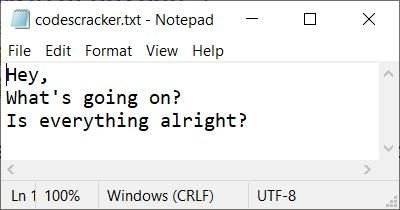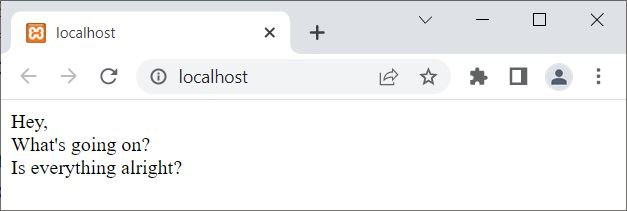The PHP function fread() is used when we need to read the content of an opened file using its pointer. For example:

```<?php
\$fp = fopen("codescracker.txt", "r");
echo \$content;
fclose(\$fp);
?>```

The output produced by the above PHP example using the fread() function is:Since I've specified 4 as the file length, read only the first 4 characters of the file. Therefore, the output produced by the above example is "Hey,". That is the first four characters of the file codescracker.txt. Here is a snapshot of the file, codescracker.txt:The syntax of the fread() function in PHP is:

`fread(filePointer, length)`

Both parameters are required. The filePointer refers to the file pointer, whereas the length parameter is used to specify the length of characters to read from the file.

If you want to read the complete content of a file, then you can use the filesize() function to calculate the size of an unknown file and apply it as the length parameter. For example:

```<?php
\$file = "codescracker.txt";
\$fp = fopen(\$file, "r");
echo \$content;
fclose(\$fp);
?>```

Now the output of the above PHP example should be:Here is the modified version of the above example:

```<?php
\$file = "codescracker.txt";
\$fp = fopen(\$file, "r");
if(\$fp)
{
echo \$content;
fclose(\$fp);
}
else
echo "<p>Unable to open the file</p>";
?>```

## PHP Read a File Line by Line

To read a file, line by line, use feof() followed by fgets() in this way:

```<?php
\$file = "codescracker.txt";
\$fp = fopen(\$file, "r");
if(\$fp)
{
while(!feof(\$fp))
{
\$line = fgets(\$fp);
echo \$line;
echo "<br>";
}
}
else
echo "<p>Unable to open the file</p>";
?>```

The output of the above PHP example should be:## PHP Read a file character by character

To read a file character by character, use feof() followed by fgetc() in this way:

```<?php
\$file = "codescracker.txt";
\$fp = fopen(\$file, "r");
if(\$fp)
{
while(!feof(\$fp))
{
\$character = fgetc(\$fp);
if(\$character == "\n")
echo "<br>";
else
echo \$character;
}
}
else
echo "<p>Unable to open the file</p>";
?>```

You will get the exact output as in the previous example.

PHP Online Test

« Previous Tutorial Next Tutorial »